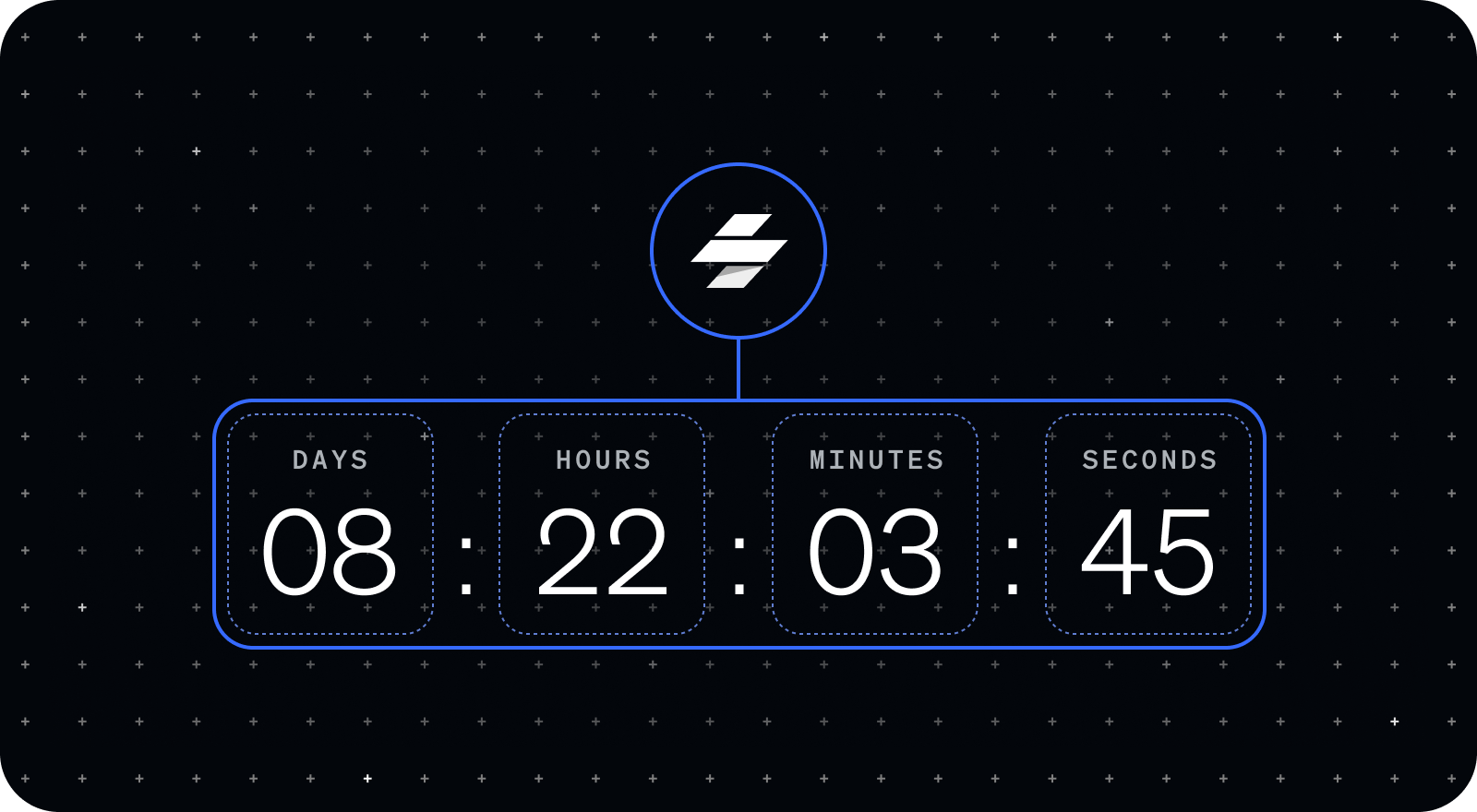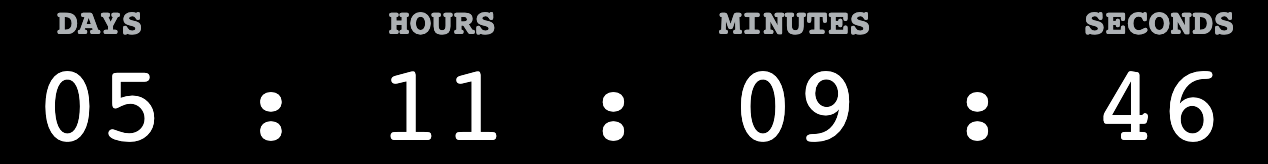In this tutorial, we are going to build a countdown tracker component using Stencil. Countdown trackers are a great way to both inform your users of an event, as well as generate excitement around the event. By the end of this tutorial you’ll have a countdown tracker component that you can reuse and customize for your own apps.

Stencil is a great tool for building reusable web components and is especially suitable for building out design systems at scale. Stencil components can be incorporated into many front-end frameworks like React, Angular, and Vue—or no framework at all. By building our countdown tracker with Stencil, we’ll have an incredibly versatile component that we can use anywhere.

Here’s the final countdown tracker component we’ll be building:You can actually see this component in action on the Ionic events site! We’re using it to count down to the Ionic Launch Event on September 28!

You can find all the code for this tutorial at the Stencil countdown tracker component GitHub repository here.

This tutorial assumes that you already have a Stencil project set up. If you don’t already have a stencil project, check out the Stencil docs to get started.

## Props and State

In order to build a countdown tracker, we need to know two critical data points: what date and time are we counting down to, and how much time is left between that time and now. The target date and time needs to be provided to us by the user, so we will accept it as a `@Prop` called `endDate` with a type of `string`. This string will need to be in ISO format so it can be converted into a JavaScript `Date` object later. We are going to display the time between the `endDate` and now in terms of days, hours, minutes, and seconds. Because these values will change over time and we want to update our countdown tracker each time they change, we need to use `@State` for each of these values. We’ll initialize them all to be “00” to start.

``````export class CountdownTracker {
@Prop() endDate: string;

@State() days: string = '00';
@State() hours: string = '00';
@State() minutes: string = '00';
@State() seconds: string = '00';
``````

## Initializing Our End Date

Because the `endDate` prop is provided as a string, we need to convert it to a JavaScript `Date` object in order to perform operations that will help us determine the time between the `endDate` and now. To do that, we can create a new `Date` object and pass it the `endDate` string we received as a property. Then, we can assign that value to a private variable called `endDateObj` so we can reference it later. We need to be sure to do this assignment in the `componentWillLoad()` lifecycle method so we have access to it when the countdown tracker is connected to the DOM.

``````private endDateObj: Date;

this.endDateObj = new Date(this.endDate);
}
``````

## Time Conversions

The core functionality of our countdown tracker is continuously calculating the time between the current date and time and the `endDate`. Fortunately, the JavaScript `Date` object has a useful function, `getTime()`, that can help us do exactly that. `getTime()` returns the number of milliseconds between the given `Date` object and January 1, 1970 (Unix Epoch). Since `getTime()` returns a number, we can use it to perform date arithmetic. We can calculate the remaining time until the `endDate` by subtracting the current time, `new Date()`, from the `endDate`. This will give us the remaining time in milliseconds, which we can convert into days, hours, minutes, and seconds.

``````tick() {
// example value: 80834343, time in milliseconds between endDate and now
const timeRemaining = this.endDateObj.getTime() - new Date().getTime();

this.seconds = Math.floor((timeRemaining / 1000) % 60)
.toLocaleString('en-US', { minimumIntegerDigits: 2 });
this.minutes = Math.floor((timeRemaining / (1000 * 60)) % 60)
.toLocaleString('en-US', { minimumIntegerDigits: 2 });
this.hours = Math.floor((timeRemaining / (1000 * 60 * 60)) % 24)
.toLocaleString('en-US', { minimumIntegerDigits: 2 });
this.days = Math.floor((timeRemaining / (24 * 60 * 60 * 1000)) % 30)
.toLocaleString('en-US', { minimumIntegerDigits: 2 });
}
``````

Let’s take a look at how we convert the `timeRemaining` in milliseconds to days, hours, minutes, and seconds. First, to convert the `timeRemaining` to seconds, we divide it by 1000 as there are 1000 milliseconds in a second. We could then convert this to minutes, by dividing by 60, but there would be some seconds remaining. Before we convert to minutes, we want to capture those remaining seconds. To do this, we can use the remainder operator to get the remaining seconds after dividing the total seconds by 60, as there are 60 seconds in a minute. A similar procedure is used for each of the other values. Use division to convert the milliseconds to the metric you are solving for, and then use the remainder operator to capture the remainder of the specific unit you are targeting.

`toLocaleString` is a convenient little method that allows us to ensure our values are represented with a minimum of two digits by prepending a ‘0’ when the value is a single digit. This way, five seconds appears as “05” instead of “5”:

``````.toLocaleString('en-US', { minimumIntegerDigits: 2 });
``````

## Create Animation Loop

We now have a function that calculates the remaining time and converts it to days, hours, minutes, and seconds. Next, we need to create a loop that will continuously run this function. For such a situation we can leverage `requestAnimationFrame()`, which allows us to make a request to the browser to execute a specific function before the next repaint. The function we want to execute is passed to `requestAnimationFrame()` as a callback. Most importantly, if that function contains another call to `requestAnimationFrame()`, it will create an animation loop. In our situation, we can create an animation loop by calling `requestAnimationFrame()` at the end of our `tick()` function and pass the `tick()` function as a callback.

``````requestAnimationFrame(this.tick.bind(this));
``````

To start our animation loop, we need to call the `tick()` function just once when the component connects to the DOM. To do that, we can use the lifecycle method `componentWillLoad()`:

``````componentWillLoad() {
this.endDateObj = new Date(this.endDate);
this.tick();
}
``````

## Time to Render

With all our necessary values calculated and updating, we can now render them on the screen.

``````render() {
return (
<Host>
<div class="column">
<p class="label">Days</p>
<p class="value">{this.days}</p>
</div>
<p class="colon">:</p>
<div class="column">
<p class="label">Hours</p>
<p class="value">{this.hours}</p>
</div>
<p class="colon">:</p>
<div class="column">
<p class="label">Minutes</p>
<p class="value">{this.minutes}</p>
</div>
<p class="colon">:</p>
<div class="column">
<p class="label">Seconds</p>
<p class="value">{this.seconds}</p>
</div>
</Host>
);
}
``````

To see our countdown tracker in action, we can use it in our `index.html` file and specify the `end-date` in ISO format.

``````<body>
<countdown-tracker end-date="2021-09-28" />
</body>
``````

You can set the `end-date` to whatever date and time you like as long as it is in ISO format.

## Stopping the Animation Loop

We’re almost there, but you may have noticed that our countdown tracker starts to behave a little funny when the `endDate` finally arrives. Instead of stopping at 00:00:00:00, the tracker continues to count down into the negatives. This is because we don’t have any condition in our `tick()` function to check if the `endDate` has been reached. As a result, our animation loop never stops. To eventually stop the loop, we need to set a `stopId` for our `requestAnimationFrame()`. This is a number that is returned by `requestAnimationFrame()` to identify the animation request. When there is no more `timeRemaining`, we stop the loop by passing that `stopId` to `cancelAnimationFrame()` and exiting from the function.

``````private stopId: number;

tick() {
// example value: 80834343, time in milliseconds between endDate and now
const timeRemaining = this.endDateObj.getTime() - new Date().getTime();

if (timeRemaining < 0) {
cancelAnimationFrame(this.stopId);
return;
}

this.seconds = Math.floor((timeRemaining / 1000) % 60)
.toLocaleString('en-US', { minimumIntegerDigits: 2 });
this.minutes = Math.floor((timeRemaining / (1000 * 60)) % 60)
.toLocaleString('en-US', { minimumIntegerDigits: 2 });
this.hours = Math.floor((timeRemaining / (1000 * 60 * 60)) % 24)
.toLocaleString('en-US', { minimumIntegerDigits: 2 });
this.days = Math.floor((timeRemaining / (24 * 60 * 60 * 1000)) % 30)
.toLocaleString('en-US', { minimumIntegerDigits: 2 });

this.stopId = requestAnimationFrame(this.tick.bind(this));
}
``````

## Add Some Styles

Finally, let’s add some styles to make our countdown tracker more visually appealing. Feel free to be creative and incorporate your own styles here.

``````@import url('https://fonts.googleapis.com/css2?family=Roboto+Mono:wght@400;700&display=swap');

:host {
font-family: 'Roboto Mono', monospace;
background-color: black;
color: white;
display: flex;
align-items: flex-end;
justify-content: space-around;
min-width: 415px;
max-width: 830px;
}

.column {
display: flex;
flex-direction: column;
align-items: center;
}

.label {
color: #d7dde2;
opacity: 0.8;
font-size: 18px;
font-weight: 700;
text-transform: uppercase;
}

.value,
.colon {
font-size: 54px;
}

p {
margin: 0;